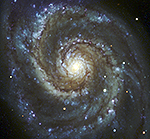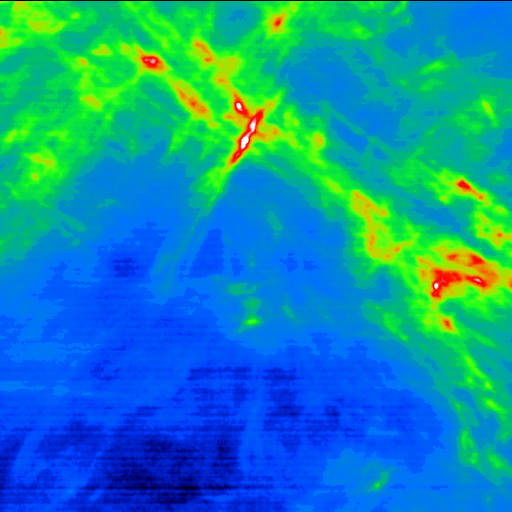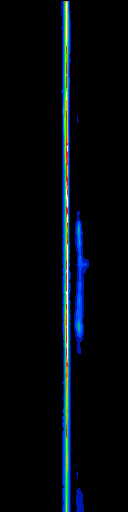Montage Montage is an astronomical image toolkit with components for reprojection, background matching, coaddition and visualization of FITS files. It can be used as a set of command-line tools (Linux, OS X and Windows), C library calls (Linux and OS X) and as Python binary extension modules.

The Montage source is written in ANSI-C and code can be downloaded from GitHub ( https://github.com/Caltech-IPAC/Montage ). The Python package can be installed from PyPI ("</i>pip install MontagePy"). The package has no external dependencies. See http://montage.ipac.caltech.edu/ for details on the design and applications of Montage.

# MontagePy.main modules: mTranspose¶

Most of Montage is focused on 2D astronomical image mosaics: reprojection, background matching, coaddition and so on. But there is also a need for tools that operate on data "cubes": three- or four-dimensional arrays where two of the axes represent the same projected sky coordinates as we find in the 2D images. Usually, the third axis is some form of wavelength.

The problem sets are not completely parallel: datacubes do not generally need background matching but you do frequently want cutouts in the none spatial dimensions and to transpose axes.

Montage includes a set of routines for manipulating datacubes:

• mmProjectCube — Reproject the spatial dimensions.
• mAddCube — Reproject the cube.
• mShrinkCube — Rescale a cube (integer scaling in the non-spatial dimensions).
• mSubCube —Cut a portion out of a cube.
• mTranspose — Transpose a cube's axes.

This routine, mTranspose, rearranges the data cube so that the axes are in new user-defined order. The primary purpose of this within Montage is to arrange the two sky spatial dimensions first so that the reprojection code (mProjectCube) will work.

Note: The MontagePy python package has no external dependencies. We include other utilities on this page to aid in visualizing MontagePy package results.

In :
from MontagePy.main import mTranspose, mViewer

help(mTranspose)

Help on built-in function mTranspose in module MontagePy.main:

mTranspose(...)
mTranspose switches around the axes for a 3D or 4D image.  This is mainly so we can get a cube rearranged so the spatial axes are the first two.

Parameters
----------
inputFile : str
Input FITS file.
outputFile : str
Output transposed FITS file.
norder : int
The number of axes (3 or 4).
order : np.ndarray
The output ordering of axes desired.
debug : int, optional
Debugging level (not for general use).

Returns
-------
status : int
Return status (0: OK, 1:ERROR).
msg : str
Return message (for errors).
mindata : float
Minimum data value in cube.
maxdata : float
Maximum data value in cube.



## mTranspose Example¶

While the most common use of mTranspose is to rearrange a datacube so the spatial dimensions come first (to allow reprojection and viewing as a sky map), our example dataset, GALFA, is already arranged like that. So our example here will show transposing away from that to a spatial-spectral view where we can view the spectrum as a function of -- in this case -- latitude.

In :
rtn = mTranspose('GALFA/shrunken/GALFA_HI_RA+DEC_012.00+10.35_N.fits',
'work/GALFA/GALFA_transpose.fits',
3, [3, 2, 1])
print(rtn)

{'status': '0', 'mindata': -1.0, 'maxdata': 45.0}


To illustrate the datacube charateristics and the effect of the above transpose, we'll show a couple of cuts throug the cube. First, in "image" mode (i.e. at cut at one particular wavelength).

In :
from IPython.display import Image

mViewer('-ct 4 -gray "GALFA/shrunken/GALFA_HI_RA+DEC_012.00+10.35_N.fits" \
-2s max gaussian-log -out work/GALFA/GALFA_HI_RA+DEC_012.00+10.35_N_transpose.png',
'', 2)

Image(filename='work/GALFA/GALFA_HI_RA+DEC_012.00+10.35_N_transpose.png')

Out:The above images is for the central wavelength of the cube, which is also the average peak wavelength for the datacube. This can be seen clearly by looking at the transpose image. Here the vertical axis is still latitude (as above) but the horizontal axis is wavelength. The signal is strongest at the central wavelength, to first order regardless of latitude (the same is true for longitude). The longitude chosen runse down the middle of the above image.

In :
mViewer('-nowcs \
-ct 4 -gray "work/GALFA/GALFA_transpose.fits" \
-2s max gaussian-log -out work/GALFA/GALFA_transpose.png',
'', 2)

print(rtn)

Image(filename='work/GALFA/GALFA_transpose.png')

{'status': '0', 'mindata': -1.0, 'maxdata': 45.0}

Out:## mTranspose Error Handling¶

If mTranspose encounters an error, the return structure will just have two elements: a status of 1 ("error") and a message string that tries to diagnose the reason for the error.

For instance, if the user references a datacube that doesn't exist:

In :
rtn = mTranspose('GALFA/shrunken/unknown.fits',
'work/GALFA/GALFA_transpose.fits',
3, [3, 2, 1])
print(rtn)

{'status': '1', 'msg': b'Input image file GALFA/shrunken/unknown.fits missing or invalid FITS'}


# Using Montage Standalone¶

### mTranspose Unix/Windows Command-line Arguments¶

mTranspose can also be run as a command-line tool in Linux, OS X, and Windows:

Usage: mTranspose [-d level] [-s statusfile] in.fits out.fits outaxis1 outaxis2 [outaxis3 [outaxis4]]

If you are writing in C/C++, mTranspose can be accessed as a library function:

/*-***********************************************************************/
/*                                                                       */
/*  mTranspose                                                           */
/*                                                                       */
/*  This module switches around the axes for a 3D or 4D image.  This is  */
/*  mainly so we can get a cube rearranged so the spatial axes are the   */
/*  first two.                                                           */
/*                                                                       */
/*  This program subsets an input image around a location of interest    */
/*  and creates a new output image consisting of just those pixels.      */
/*  The location is defined by the RA,Dec (J2000) of the new center and  */
/*  the XY size in degrees of the area (X and Y) in the direction of     */
/*  the image axes, not Equatorial coordinates.                          */
/*                                                                       */
/*   char  *inputFile      Input FITS file                               */
/*   char  *outputFile     Subimage output FITS file                     */
/*                                                                       */
/*   int    norder         Number of axes (3 or 4)                       */
/*                                                                       */
/*   int    order          The output ordering of axes desired.  For     */
/*                         instance, to convert a cube where the first   */
/*                         axis is wavelength, the second RA and the     */
/*                         third Dec to RA, Dec, wavelength ordering     */
/*                         (a common situation), the output order is     */
/*                         2,3,1.                                        */
/*                                                                       */
/*   int    debug          Debugging output level                        */
/*                                                                       */
/*************************************************************************/

struct mTransposeReturn *mTranspose(char *inputFile, char *outputFile, int innorder, int *inorder, int debug)


Return Structure

struct mTransposeReturn
{
int    status;        // Return status (0: OK, 1:ERROR)
char   msg ;    // Return message (for error return)
char   json;    // Return parameters as JSON string
double mindata;       // Minimum data value.
double maxdata;       // Maximum data value.
};